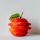Home
IT Knowledge
Inspiration
Languages
EN

# Python - check if string is empty

0 points
Created by:Khloe-Kaiser
398

In this article, we would like to show you how to check if string is empty in Python.

Quick solution:

``````text = ""
print(bool(text))  # False``````
``````text = "abc"
print(bool(text))  # True``````

Note:

In Python empty strings are considered as `false`.

## Practical example

In this example, we create a simple `if` statement to check if the string is empty or not.

Case 1: empty string

``````text = ""

if not text:
print("true")
else:
print("false")``````

Output:

``true``

Case 2: not empty string

``````text = "abc"

if not text:
print("true")
else:
print("false")``````

Output:

``false``

Note:

In this example, we don't need `print()` function because the expression itself returns `true` or `false`. It can be replaced with some action.